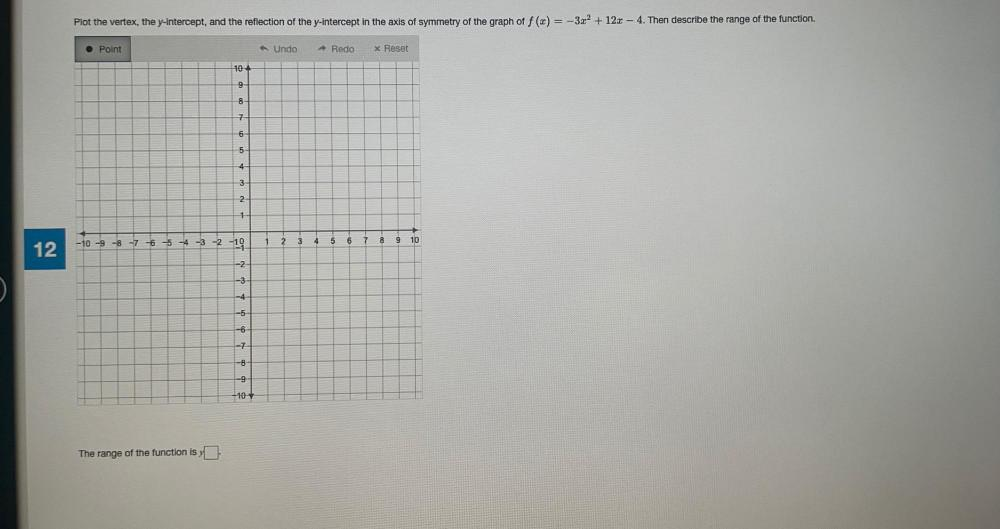Question:

# Plot the vertex, the y-intercept, and the reflection of the y-intercept in the axis of symmetry of the graph of f(x) = -3x² + 12Plot the vertex, the y-intercept, and the reflection of the y-intercept in the axis of symmetry of the graph of f(x) = -3x² + 12x - 4. Then describe the range of the function. Point Undo Redo x Reset 104 9 8 7 6 5 4 3 2 1 2 3 4 5 6 7 8 9 10 12 -10 -9 -8 -7 -6 -5 -4 -3 -2 -19 -2 -3 -4 -5 -6 -7 -9 10 The range of the function is